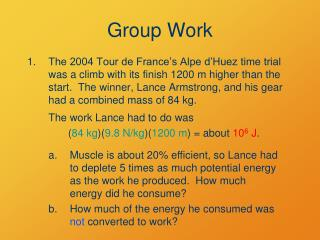DownloadDownload PresentationGroup Work

# Group Work

Télécharger la présentation## Group Work

- - - - - - - - - - - - - - - - - - - - - - - - - - - E N D - - - - - - - - - - - - - - - - - - - - - - - - - - -
##### Presentation Transcript

1. Group Work • The 2004 Tour de France’s Alpe d’Huez time trial was a climb with its finish 1200 m higher than the start. The winner, Lance Armstrong, and his gear had a combined mass of 84 kg. The work Lance had to do was (84 kg)(9.8 N/kg)(1200 m)= about106 J. Muscle is about 20% efficient, so Lance had to deplete 5 times as much potential energy as the work he produced. How much energy did he consume? How much of the energy he consumed was not converted to work?

2. Heat and Phase Changes Our interaction with the world of the small

3. Announcements • Questions on drill?

4. What’s the Point? • What happens to all our energy? • How does energy convert in freezing, melting, evaporation, and condensation?

5. Objectives • Define and differentiate heat and temperature. • Explain the role of heat in conservation of energy. • Describe the energy transfer of phase changes.

6. CPS Question • Transfer of force. • Transfer of momentum. • Transfer of mass. • Transfer of energy. What is work?

7. Mechanical Equivalent of Heat James Joule’s life-long obsession Identical effects of adding heat to a system and doing work on it. Source: Griffith, The Physics of Everyday Phenomena

8. Heat and work • Doing work on an object changes its energy. • Heat transfer is another way to change an object’s energy! • Work heat: easy • Heat  work: more difficult

9. CPS Question • Its speed increases. • Its height increases. • Its temperature increases. • Its energy does not change. When heat is added to an object, how does the object’s energy change?

10. Temperature and Energy • Averagetranslational molecular kinetic energy is 1/2 kBT per mode of motion. • kB = 1.3806610–23 J/K (Boltzmann constant) • Individual molecules can have higher or lower kinetic energies than average.

11. Terms • Temperature is proportional to average molecular translational kinetic energy. • Internal energy (U) is total molecular kinetic + potential energy. • Heat is transferred molecular energy.

12. When a red-hot piece of iron is dropped into a bucket of water, the water CPS Question becomes hotter. increases its temperature. increases its internal energy. receives heat from the iron. all of the above.

13. c = q mDT Specific Heat (Capacity) • Heat needed to change the temperature of a unit amount of a substance. • q= heat input • m = mass of sample • Dt = temperature change • Units: J/(kg K) or cal/(kg K) • Intensive

14. Heat Capacity • Heat needed to change the temperature of a particular sample. C = q/DT = mc • Units: J/K • Extensive

15. Group Work • Show that the temperature change DT of an object to which an amount of heat q is added is given by the formula DT = q/(mc) where m is the object’s mass and c is its specific heat. Hint: What is the formula for specific heat?

16. Group Work • The specific heat of Lance Armstrong is about the same as the specific heat of water: 4184 J/(kg °C). If all the non-work energy he converted in the Alpe d’Huez climb stayed in his 75-kg body, how much would his body temperature have risen?

17. Heat Units • Joule • Calorie (cal): heat needed to raise 1 gram of water 1 degree C (or K) = 4.184 J. • British Thermal Unit (BTU): heat needed to raise 1 pound of water 1 degree F = 1054.35 J

18. Another Heat Unit • U.S. Food Calorie: Cal = 1000 cal • Food energy values are often presented in kJ in other countries

19. Quick Group Work • How many Calories (food calories) did Lance burn in the Alpe d’Huez climb?

20. Phase Changes

21. Phase Changes • Melting, boiling, freezing, condensing… • Added or removed heat changes the substance’s potential rather than kinetic energy • Water freezes at 0 °C, boils at 100 °C (well, at 92 °C in Laramie) • Not all heat transfer is expressed as a temperature change.

22. Molecular simulations • Water phase changes

23. Evaporation of a Liquid • More energetic jostling = higher temperature • An especially fast molecule at the surface may detach!

24. Evaporation of a Liquid • More energetic jostling = higher temperature • An especially fast molecule at the surface may detach!

25. KE PE Evaporation • Evaporating molecules carry away energy • Remaining liquid cools (KE decreases)

26. CPS Question When are the molecules’ potential energies highest? • When they are together in the liquid. • When they are separated in the gas.

27. Phase Changes • Potential energies: Solid < Liquid <Gas • During a phase change, potential energy, not kinetic energy (temperature) changes. • Heating or cooling a changing phase does not change its temperature!

28. steam Water boils Liquid water Ice melts ice Heating Curve for Water

29. Latent heat • Potential energy of phase change (energy required to change the phase of 1 kg of substance) • Water’s latent heat of fusion (melting): 335,000 J/kg • Water’s latent heat of vaporization: 2,255,000 J/kg

30. Group Work • During the Alpe d’Huez climb, how much sweat would Lance have needed to evaporate to keep his body temperature constant? The heat q needed to vaporize a mass m of water is q = m (2.255 106 J/kg). Solve for mass m and substitute in the values.

31. Reading for Next Time Thermodynamics Big ideas: Why most processes are irreversible Entropy